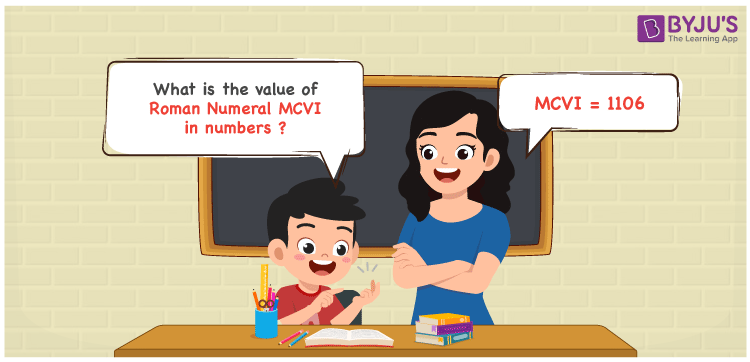Checkout JEE MAINS 2022 Question Paper Analysis : Checkout JEE MAINS 2022 Question Paper Analysis :

# MCVI Roman Numerals

MCVI Roman Numerals is 1106. The system of expressing numbers using the Roman alphabet is known as Roman Numerals. To learn the technique of expressing Roman Numerals in a precise manner, students can access Roman Numerals in PDF format anytime for free. In this article, we will discuss how to express the number form of the Roman Numeral MCVI.

 Number Roman Numeral 1106 MCVI

## How to Write MCVI Roman Numerals?We use the following methods to represent the numerical value of MCVI Roman Numerals

First Method:

• Break the Roman Numerals into single letters
• MCVI = M + C + V + I
• Write the numerical value of each letter and add/subtract them
• MCVI = 1000 + 100 + 5 + 1 = 1106

Second Method:

In this method, we consider the groups of Roman Numerals for addition or subtraction

• MCVI = M + C + VI = 1000 + 100 + 6 = 1106
• Therefore the numerical value of MCVI Roman Numerals is 1106

## Video Lesson on Roman Numerals## Related Articles

Roman Numerals 1 to 50

Roman Numerals 1 to 100

Roman Numerals 1 to 1000

Roman Numerals Conversion

L Roman Numerals

Roman Numeral XXXIX

## Frequently Asked Questions on MCVI Roman Numerals

### What is the numerical value of MCVI Roman Numerals?

The numerical value of MCVI Roman Numerals 1106.

### What is the value of (99 – 86) + 1106 in Roman Numerals?

(99 – 86) + 1106 = 1119. The number 1119 in Roman Numerals is MCXIX. Hence the value of (99 – 86) + 1106 in Roman Numerals is MCXIX.

### What number should be added to 1000 to get 1106? Express the answer in Roman Numerals.

1106 – 1000 = 106. The number 106 should be added to 1000 to get 1106. Thus the number 106 in Roman Numerals is CVI.